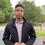# Physics Discussiom questions

$1$A ball rolls without slipping on a perfectly flat plane of infinite extent. Will it roll forever? If not, what would stop it?

$2$ Since only changes in potential energy are important in any problem, a student decides to let the elastic potential energy of a spring be zero when the spring is stretched a distance of x1. The student decides therefore to let U={1/2}k(x-x1)^2. Is this correct?”)

$3$.would space be effected by infinite amount of gravity? we know space has no mass and this might be a wrong question to ask. space is expanding because of dark energy, can it contract because of an infinite amount of gravity. ) -(This is not my homework just discussion question I found)Note by Mardokay Mosazghi
7 years, 3 months ago

This discussion board is a place to discuss our Daily Challenges and the math and science related to those challenges. Explanations are more than just a solution — they should explain the steps and thinking strategies that you used to obtain the solution. Comments should further the discussion of math and science.

When posting on Brilliant:

• Use the emojis to react to an explanation, whether you're congratulating a job well done , or just really confused .
• Ask specific questions about the challenge or the steps in somebody's explanation. Well-posed questions can add a lot to the discussion, but posting "I don't understand!" doesn't help anyone.
• Try to contribute something new to the discussion, whether it is an extension, generalization or other idea related to the challenge.

MarkdownAppears as
*italics* or _italics_ italics
**bold** or __bold__ bold
- bulleted- list
• bulleted
• list
1. numbered2. list
1. numbered
2. list
Note: you must add a full line of space before and after lists for them to show up correctly
paragraph 1paragraph 2

paragraph 1

paragraph 2

[example link](https://brilliant.org)example link
> This is a quote
This is a quote
    # I indented these lines
# 4 spaces, and now they show
# up as a code block.

print "hello world"
# I indented these lines
# 4 spaces, and now they show
# up as a code block.

print "hello world"
MathAppears as
Remember to wrap math in $$ ... $$ or $ ... $ to ensure proper formatting.
2 \times 3 $2 \times 3$
2^{34} $2^{34}$
a_{i-1} $a_{i-1}$
\frac{2}{3} $\frac{2}{3}$
\sqrt{2} $\sqrt{2}$
\sum_{i=1}^3 $\sum_{i=1}^3$
\sin \theta $\sin \theta$
\boxed{123} $\boxed{123}$

Sort by:

1. Rolling resistance would stop it. In short, the net normal force will not act exactly on the center of mass and would produce a small torque which would eventually stop the ball rolling.

2. No, its a baseless assumption. The given formula is derived by integrating very small potential energies and is only valid when spring's initial position is at x = 0.

3. No I think the space would expand. Is there any special reason why you have chosen infinite gravity? You could have simply asked if gravity would conract the space or not. Why infinite gravity my friend?

Ask if you doubt any of this.

New Question 4: What would be the direction of frictional force on a rolling without slipping ball? And why? If you think it's in the backward direction, why don't the ball accelerates? If you think it's in front direction why don't the ball disaccelerates like a cube if thrown forward with the same speed?

- 7 years, 2 months ago

I don't seem to find the answer do you know the answer

- 7 years, 2 months ago

1.Yes because of gravity

- 7 years, 2 months ago

1 the ball will stop because of friction

3 scientist also thik that universe will colapse because of graviti of every galaxy but it is not happening so the energy  that is acting in the univers Is known as dark energy because we dont know about it

- 6 years, 11 months ago